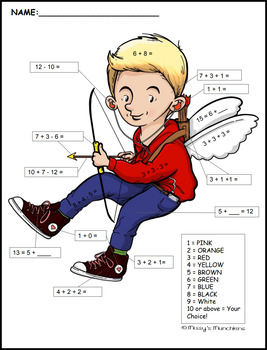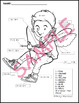# Valentine Color By Number ADDITION & SUBTRACTION Operations & Algebraic ThinkingSubject
Grade Levels
Resource Type
Common Core Standards
Product Rating
File Type

Compressed Zip File

Be sure that you have an application to open this file type before downloading and/or purchasing.

334 KB|3 pages
Share
Product Description

You are purchasing a Valentine themed modern cupid Color by Number PDF printable worksheet. You will be given two keys in the zip file - a BW version with the problems solved and a color version without the problems solved.

This Color By Number is Common Core Aligned.

The skill most covered in this worksheet is Operation and Algebraic Thinking.

Students will use Commutative property of addition; ex: If 8 + 3 = 11 is known, then 3 + 8 = 11 is also known.

Students will use Associative property of addition; ex: To add 2 + 6 + 4, the second two numbers can be added to make a ten, so 2 + 6 + 4 = 2 + 10 = 12.

Students will Determine the unknown whole number in an addition or subtraction equation relating three whole numbers. ex: Determine the unknown number that makes the equation true in each of the equations 8 + ? = 11, 11 = 13 - ?

I hope your students enjoy this fun math Color By Number!

Happy Valentine's Day

Graphics by:

35 Corks Art Studio

Creative Clips - Krista Wallden

Total Pages
3 pages
Answer Key
Included
Teaching Duration
N/A
Report this ResourceSign Up